## SQL Bridge Tool to Exploratory Data Analysis

edaSQL is a library to link SQL to Exploratory Data Analysis and further more in the Data Engineering. This will solve many limitations in the SQL studios available in the market. Use the SQL Query language to get your Table Results.

## Installation

Install dependency Packages before installing edaSQL

```pip install pyodbc
pip install ipython```

Optional dependency for better visualization – Jupyter Notebook

`pip install notebook`

Now Install using pip . Offical Python Package Here!!

`pip install edaSQL`

(OR)

Clone this Repository. Run this from the root directory to install

`python setup.py install`

## Documentation## Need help?

Stuck on your edaSQL code or problem? Any other questions? Don’t
hestitate to send me an email ([email protected]).

## edaSQL Jupyter NoteBook Tutorial

Access the sample Jupyter Notebook here!!

Access the Sample Data Used in this Repo

edaSQL for DataFrame: If you are using the CSV or Excel as a source , Read using the Pandas & start from the 3. Data Overview

### Import Packages

```import edaSQL
import pandas as pd```

### 1. Connect to the DataBase

```edasql = edaSQL.SQL()
sqlDriver='ODBC Driver 17 for SQL Server')```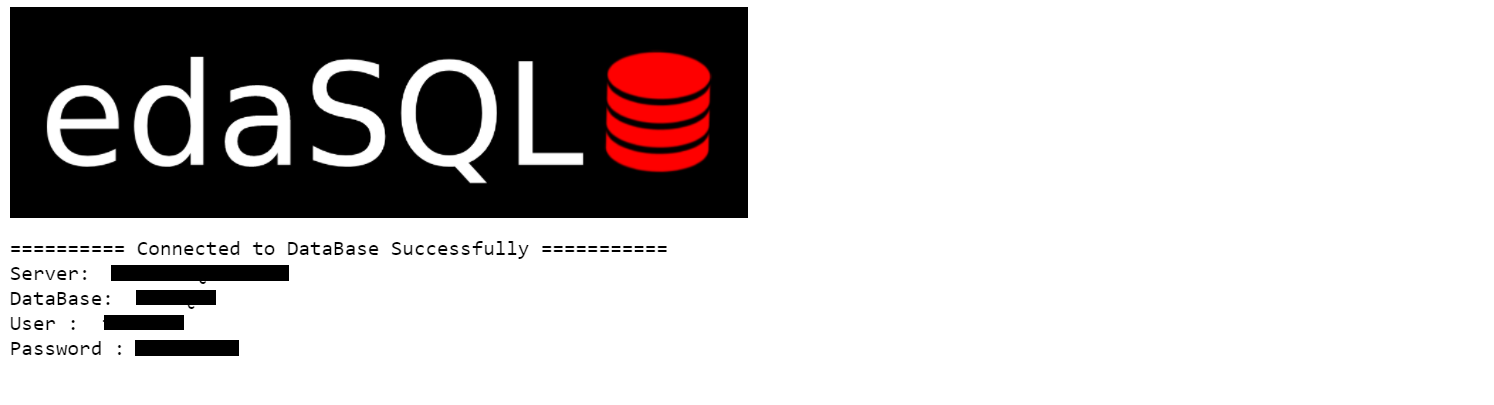### 2. Query Data

```sampleQuery = "select  * from INX"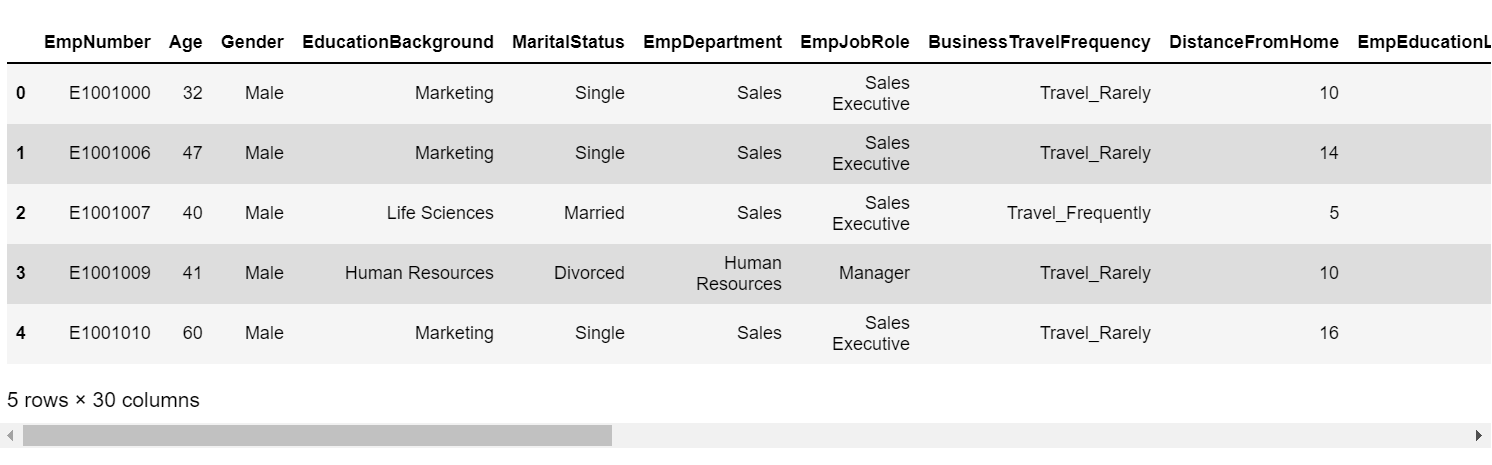### 3. Data Overview

```insights =  edaSQL.EDA(dataFrame=data,HTMLDisplay=True)
dataInsights =insights.dataInsights()```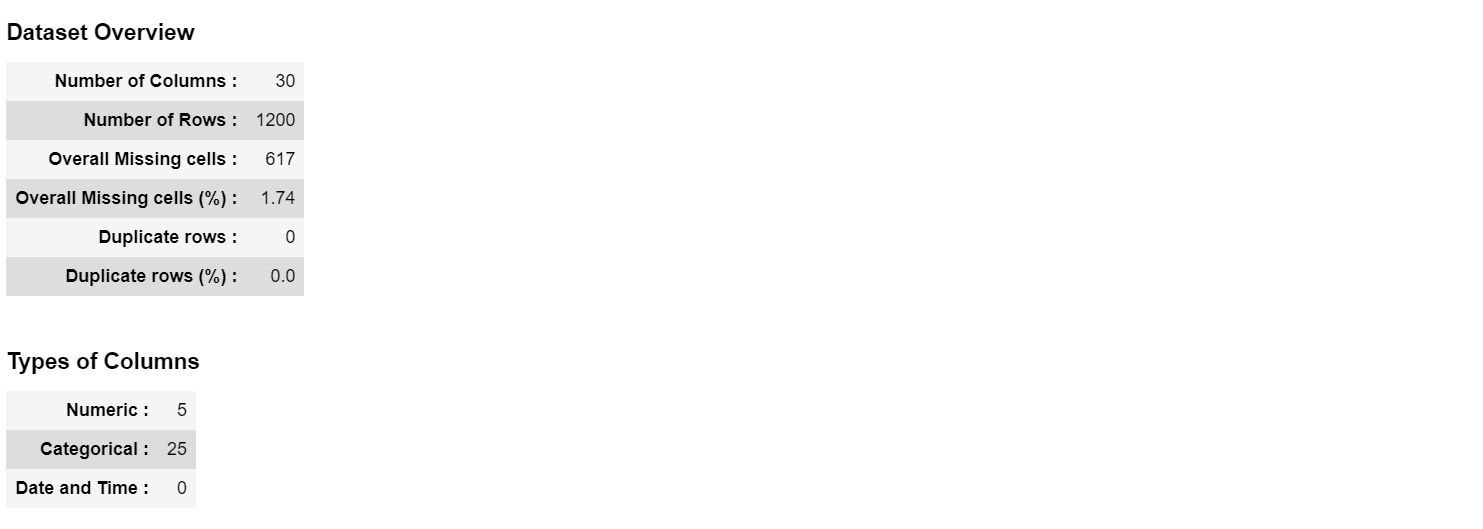`deepInsights = insights.deepInsights()`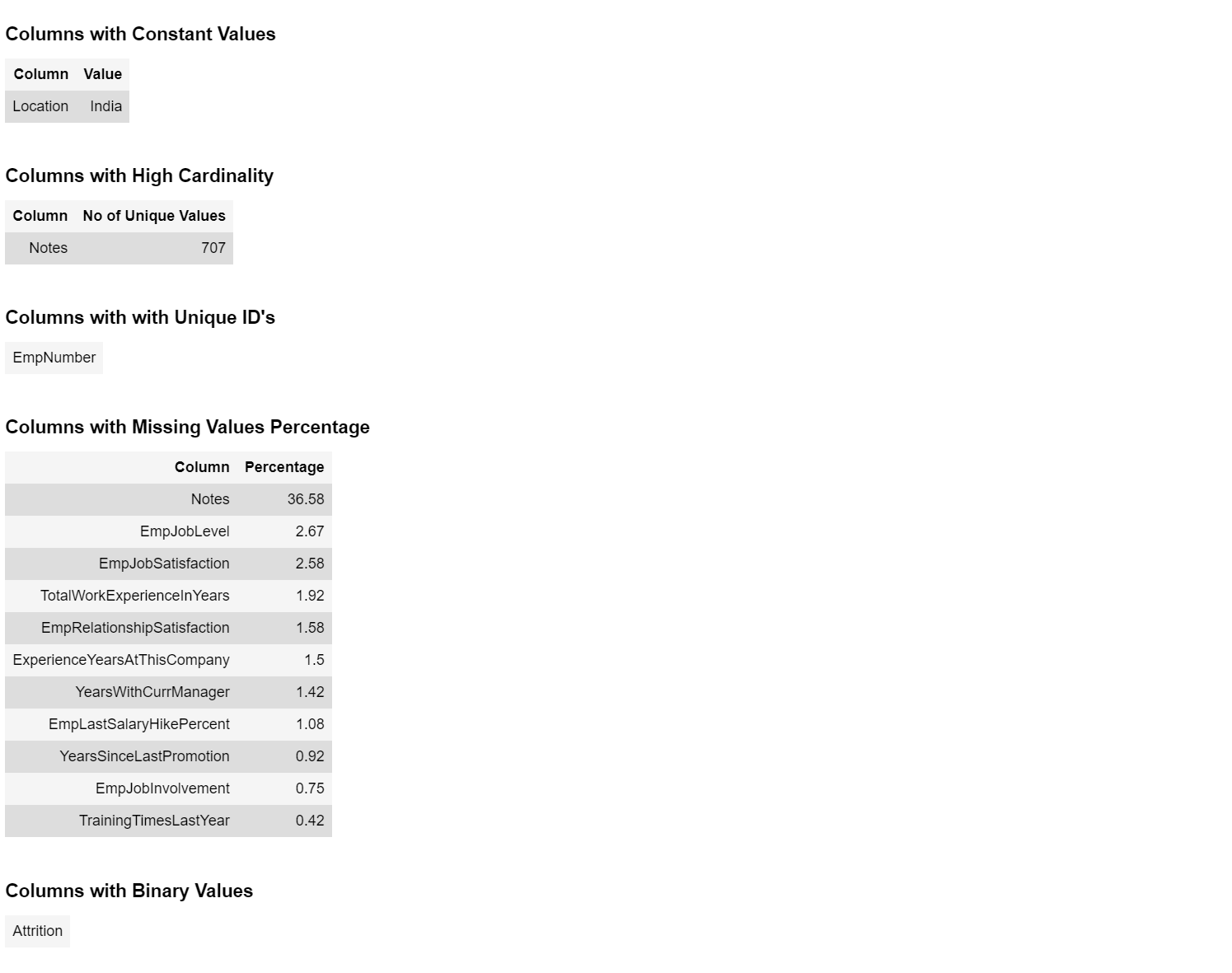### 4. Correlation

```eda = edaSQL.EDA(dataFrame=data)
eda.pearsonCorrelation()```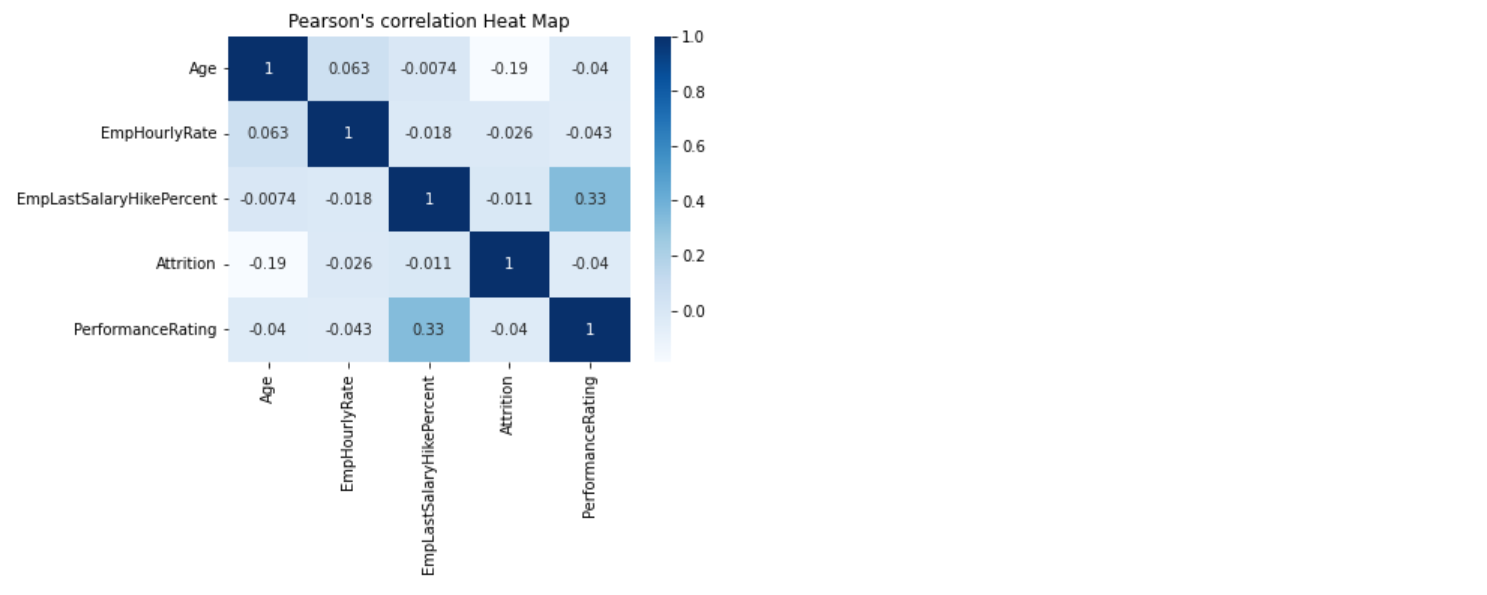`eda.spearmanCorrelation()`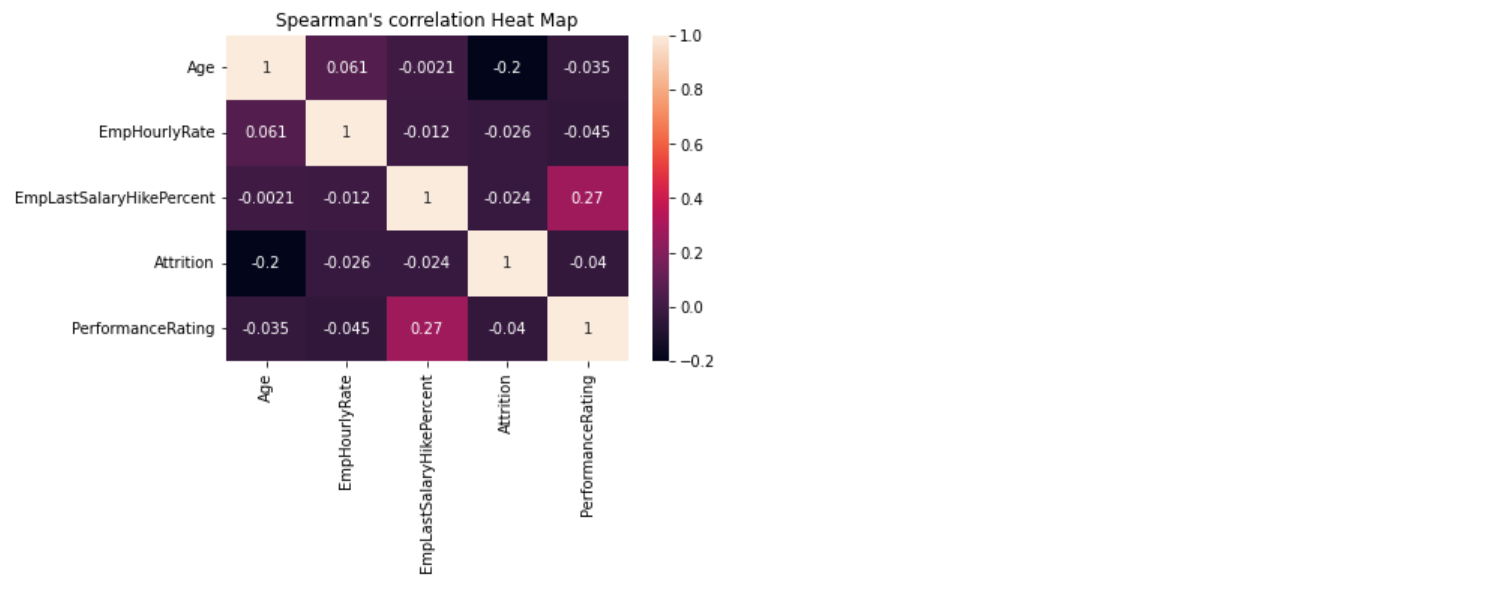`eda.kendallCorrelation()`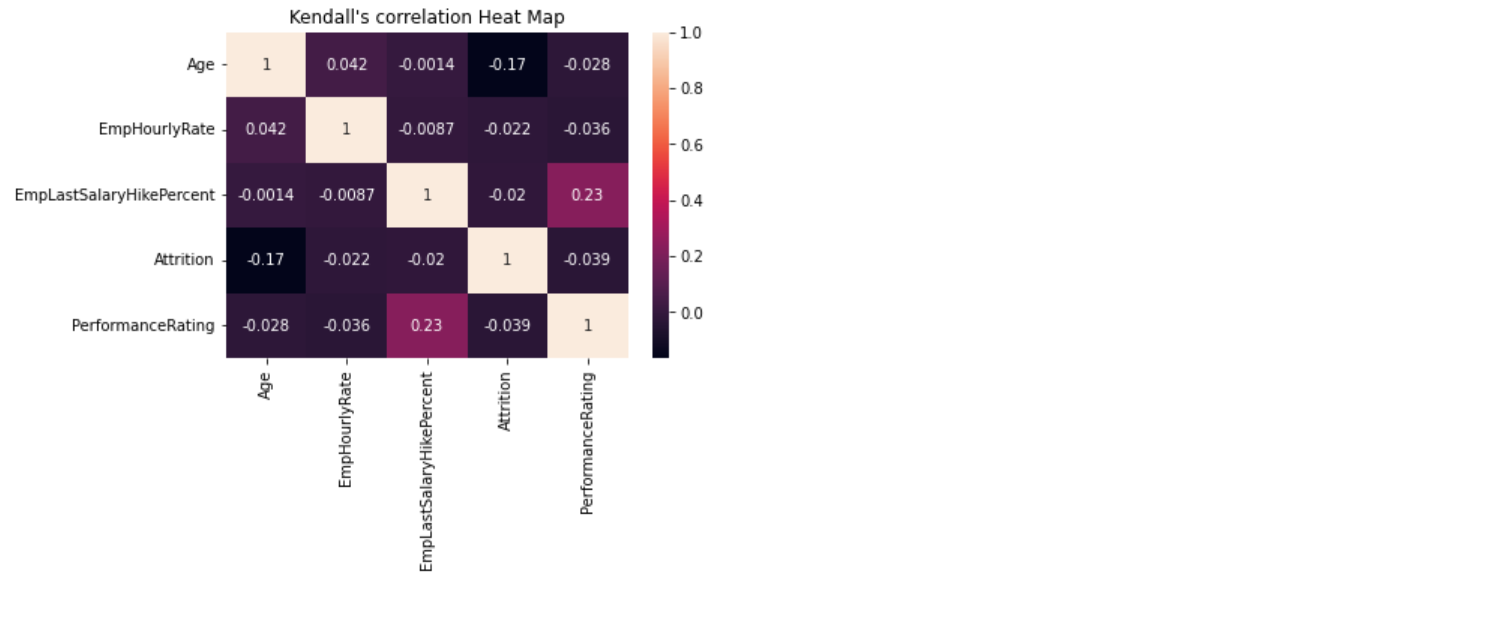### 5. Missing Values

`eda.missingValuesPlot(plot ='matrix')`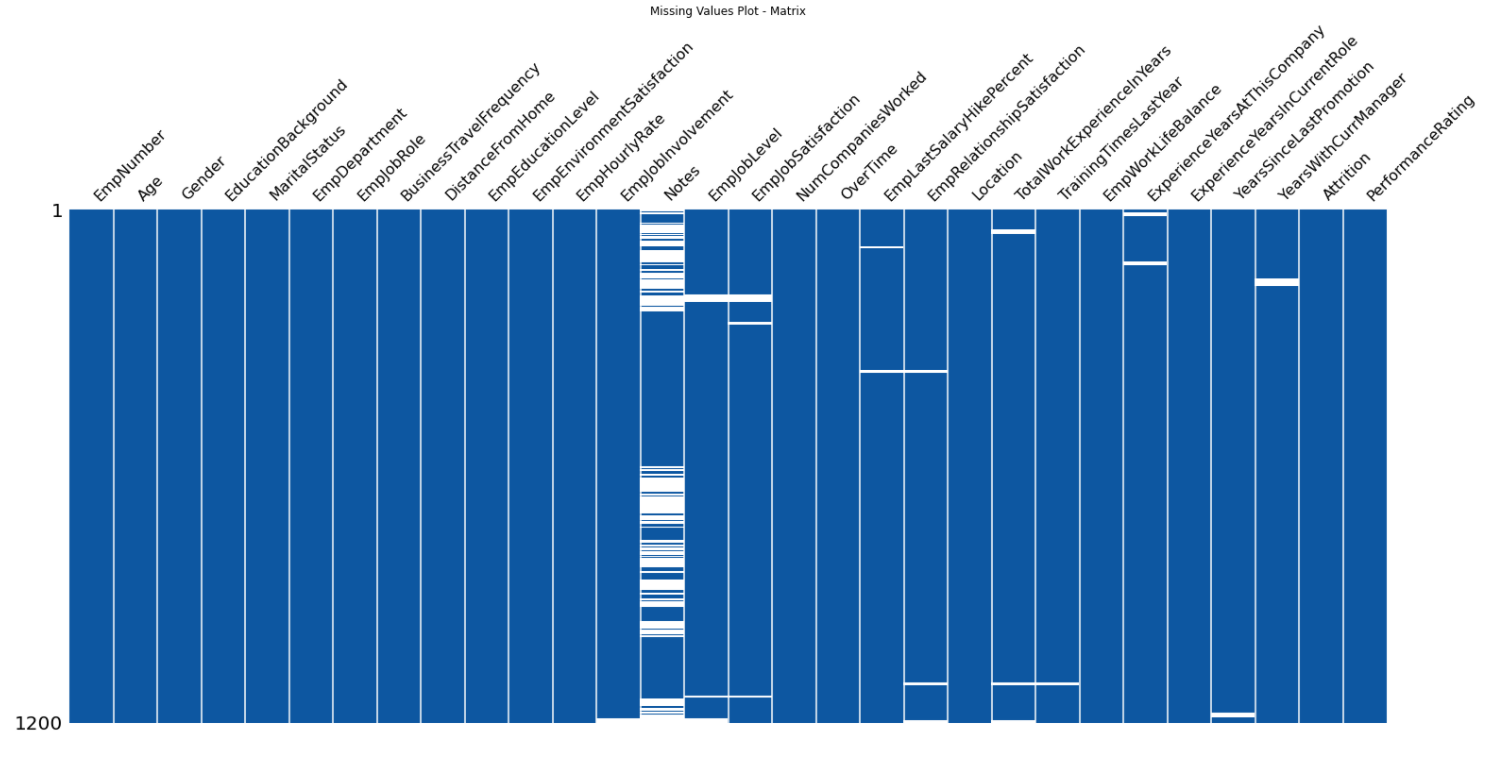`eda.missingValuesPlot(plot ='bar')`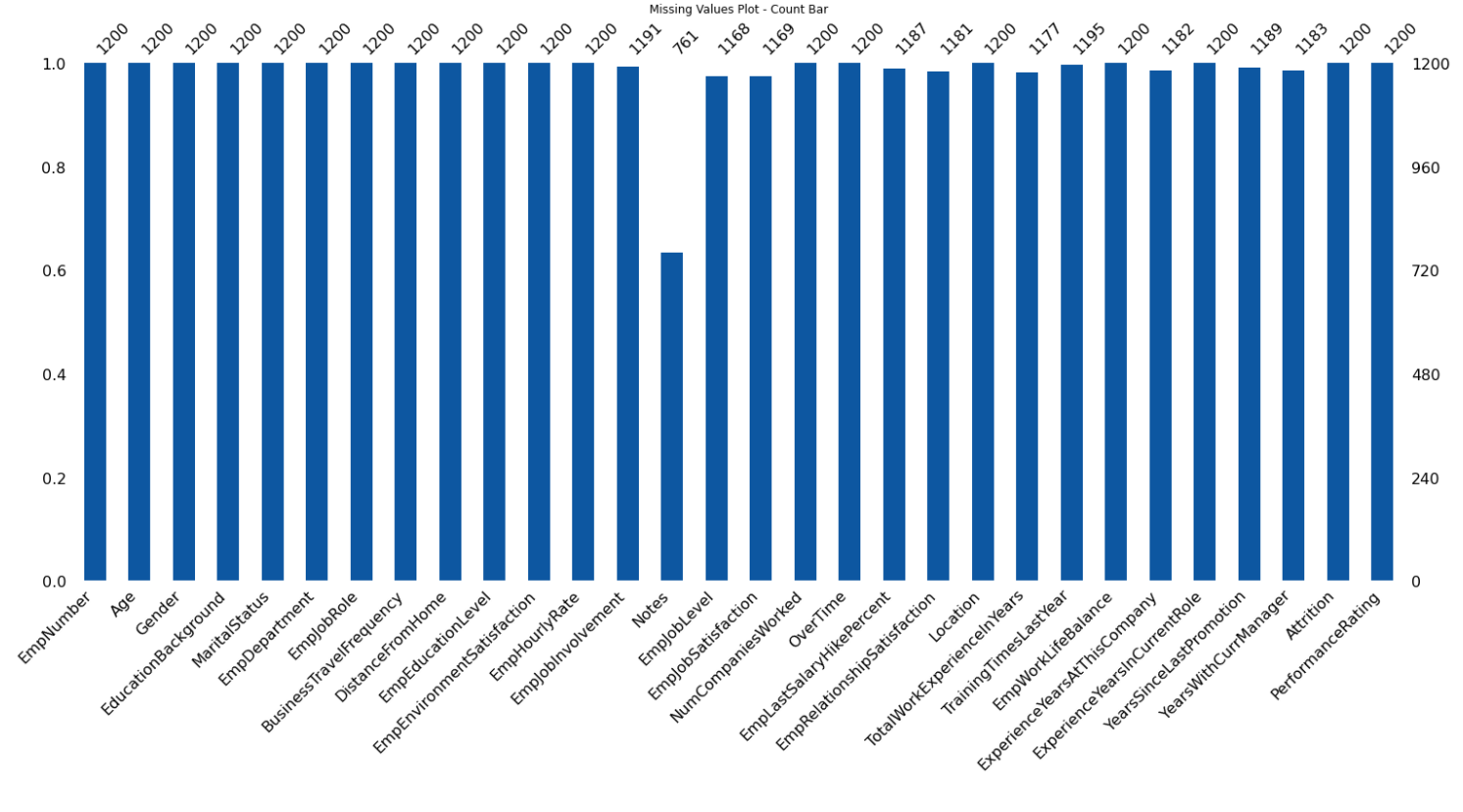`eda.missingValuesPlot(plot ='heatmap')`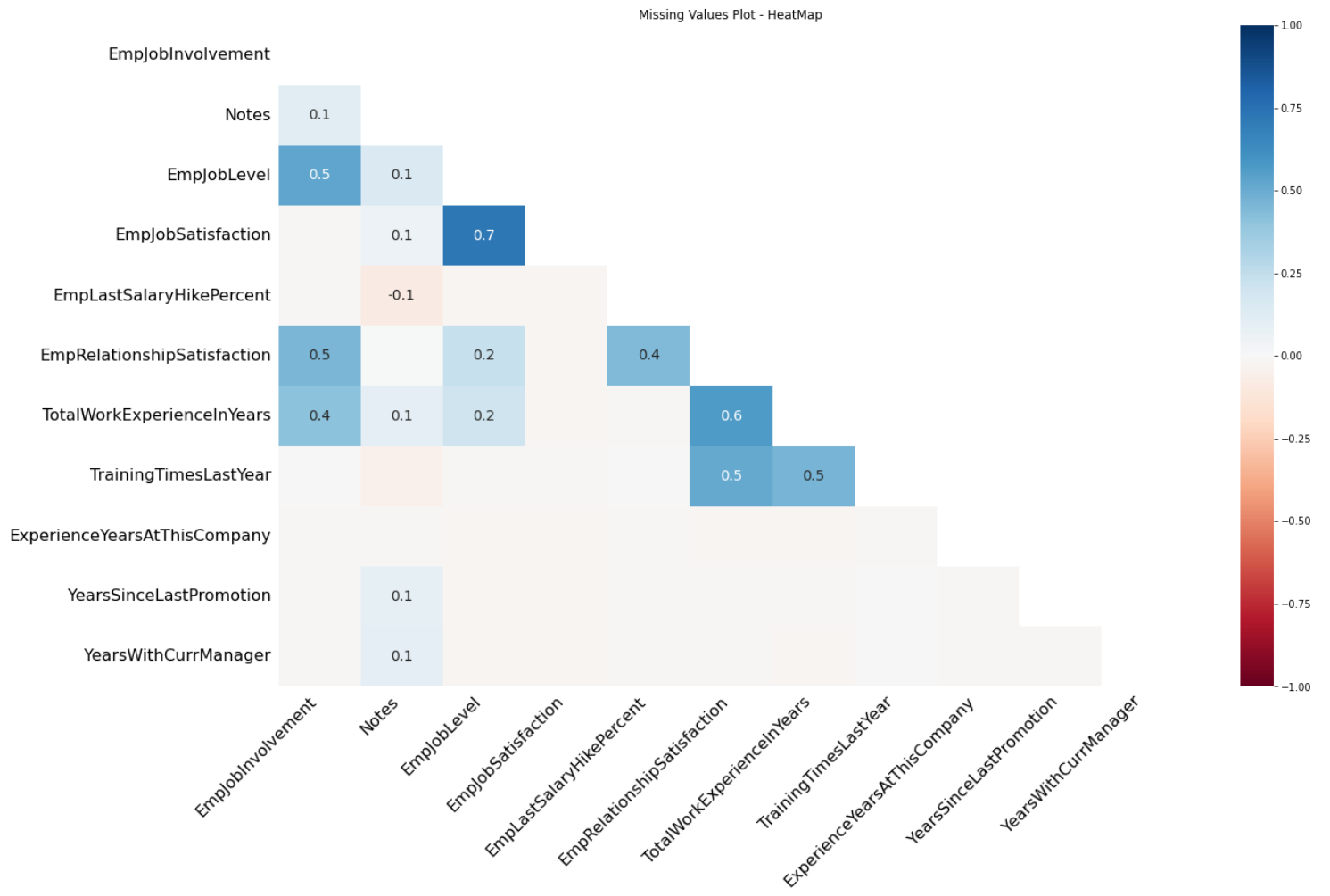`eda.missingValuesPlot(plot ='dendrogram')`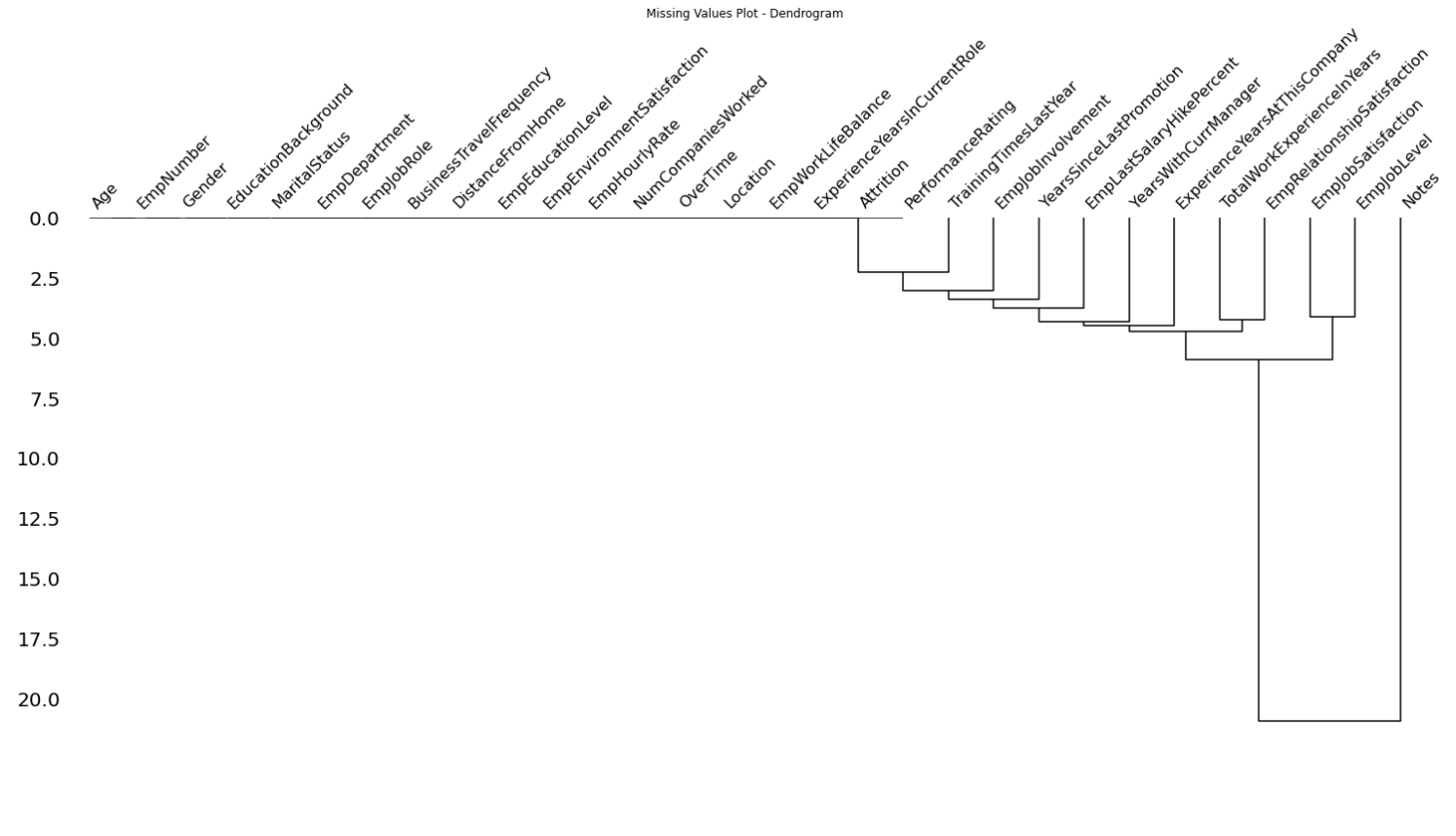### 6. Outliers

`eda.outliersVisualization(plot = 'box')`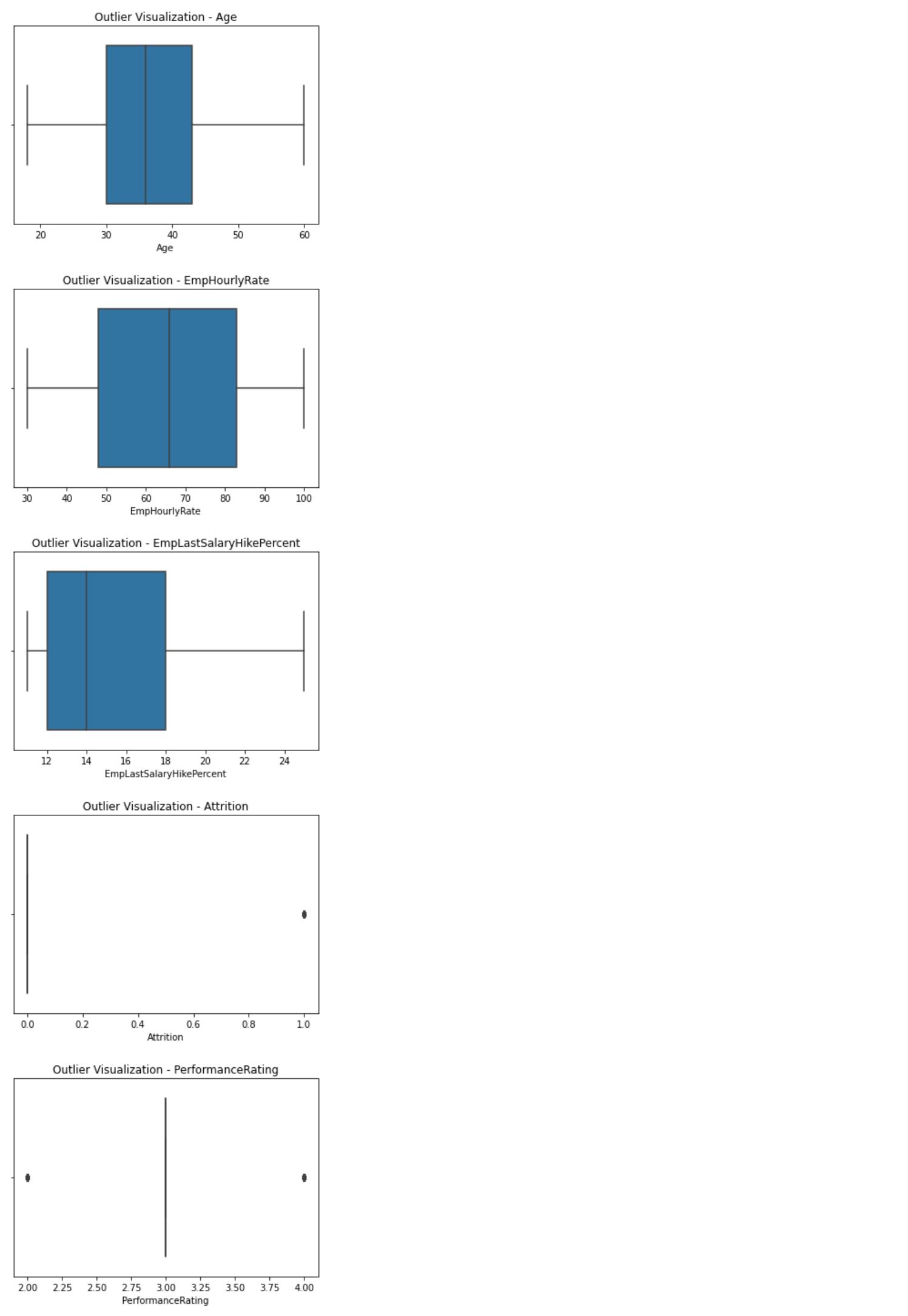`eda.outliersVisualization(plot = 'scatter')`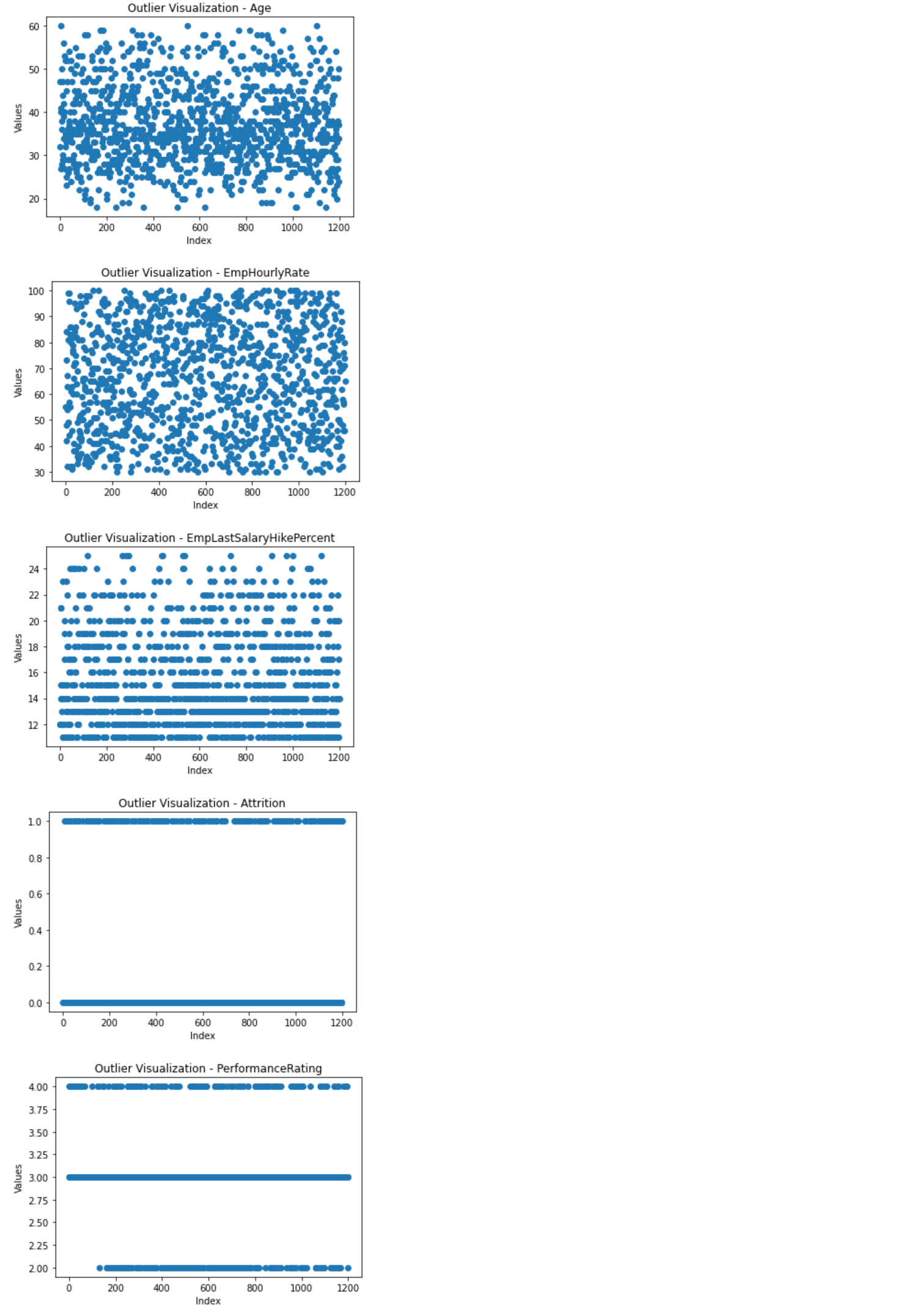`outliers = eda.getOutliers()`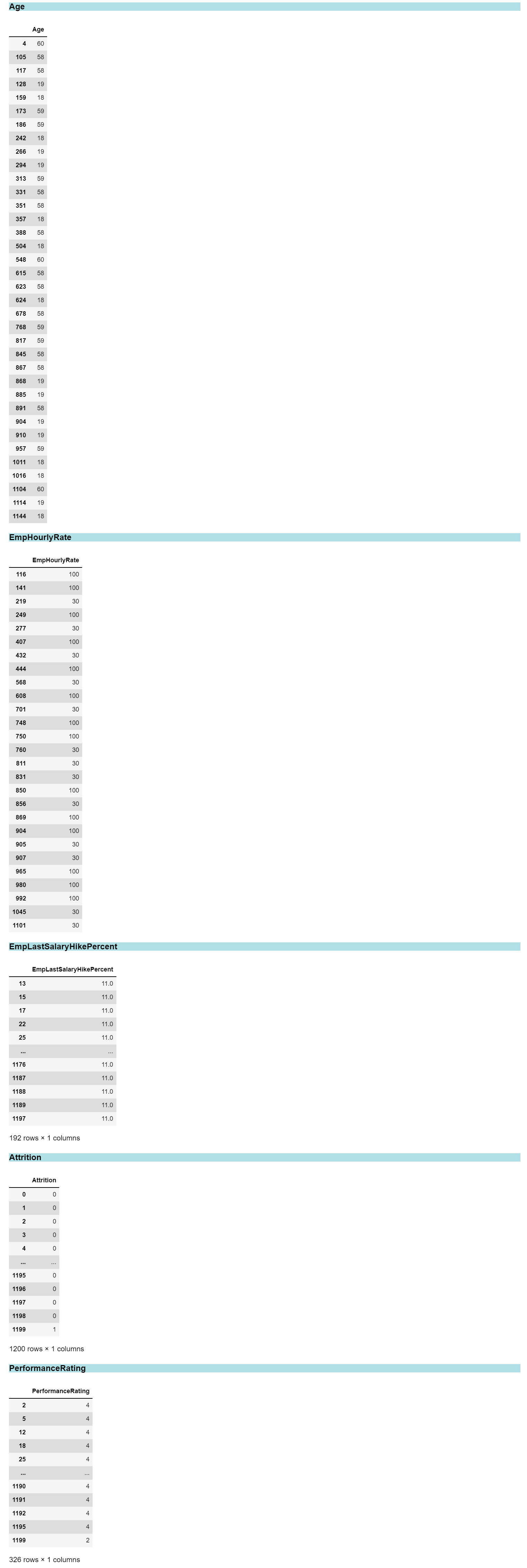View Github